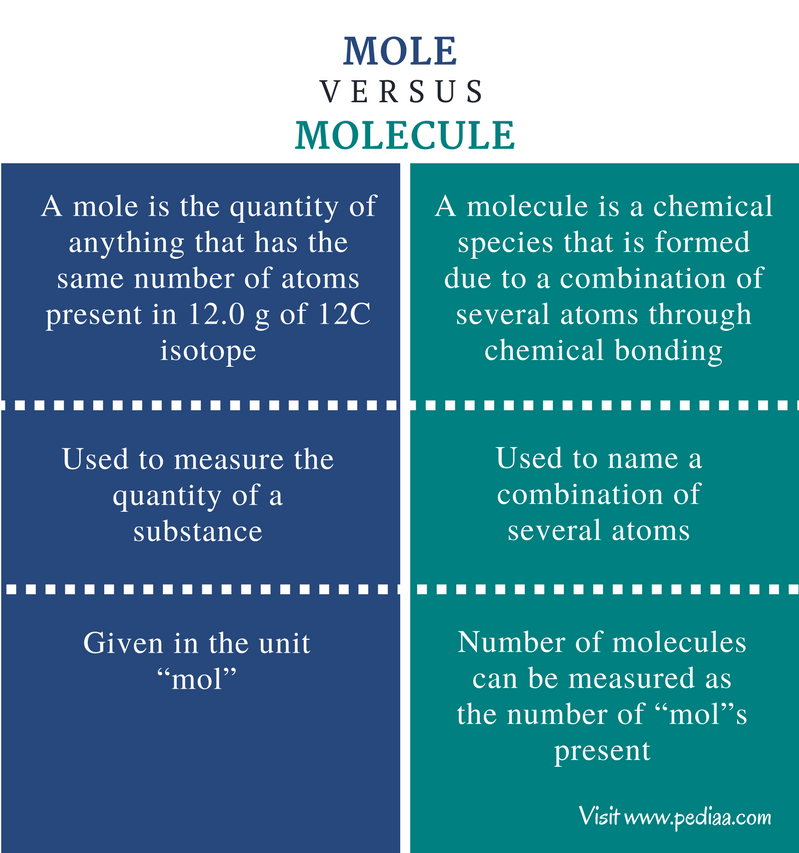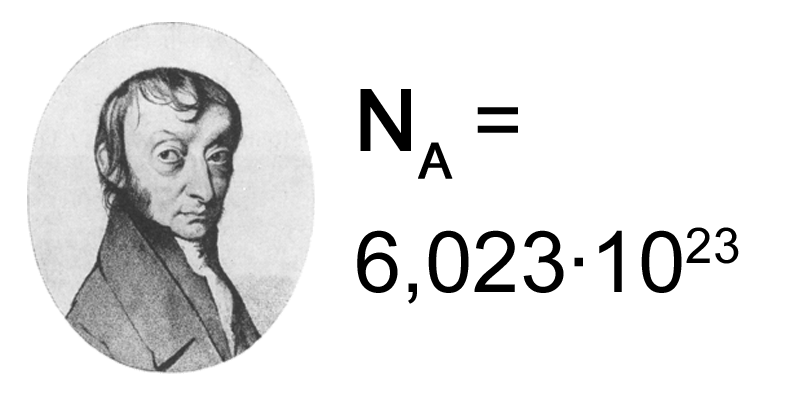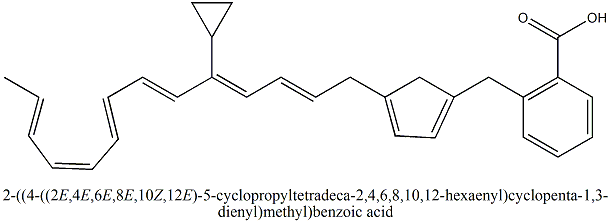# Difference Between Mole and Molecule

## Main Difference – Mole vs Molecule

Mole and molecule are two distinct terms used in chemistry. The term mole is used to represent an amount that cannot be measured with units like grams or milligrams. Therefore, a mole measures the number of atoms, ions or molecules. A molecule is a chemical species that is formed due to the combination of several atoms through chemical bonding. A molecule does not carry any electrical charge. The atoms that build up a molecule can be of the same element or different elements. The ratio between these atoms is different from one molecule to another. The number of molecules present in a system can be measured in moles. The main difference between mole and molecule is that mole is a unit of measurement of quantity whereas molecule is a chemical species that is made out of atoms.

### Key Areas Covered

1. What is a Mole
– Definition, Historical Background, Applications
2. What is a Molecule
– Definition, Types, and Uses
3. What is the Difference Between Mole and Molecule
– Comparison of Key Differences

Key Terms: Atom, Avogadro’s Number, Ion, Mole, Molecule## What is a Mole

A mole is the quantity of anything that has the same number of atoms present in 12.0 g of 12C isotope. The value of a mole is given as 6.023 x 1023. This value is called Avogadro’s number. That means, 12.0 g of 12C isotope is composed of 6.023 x 1023 carbon atoms. In other words, a mole of a carbon is composed of one Avogadro number of carbon atoms.The number of moles can be given by the unit “mol”. The term mole can be used with any chemical species such as atoms, molecules, ions, etc. Therefore, one mole of sulfur means 1 mol of sulfur atoms. One mole of carbon dioxide means, 1 mol of CO2 molecules.

When it comes to the atomic level, units like grams or milligrams are not that much useful since we need to measure very minute amounts. In 1805, John Dalton came up with the first standard atomic weight table which was composed of the atomic weight of each element with respect to hydrogen. The atomic weight of hydrogen was taken as 1. Therefore, these were relative atomic masses. Later, with the development of atomic theory, the concept of mole was introduced by Wilhelm Ostwald, a German scientist. Since then, mole became a basic SI unit that is symbolized by “mol.”

Mole is a commonly used term in chemical reactions. The amount of product that can be obtained by a certain amount of reactants can be predicted by determining the mole ratios between reactants and products of a chemical reaction. The atomic weight is given as the mass of one mole of that element. If not, it is very difficult to handle the weight of atoms since it is a very minute value.

For example,

The weight of one atom of oxygen                = 2.6 x 10-26 kg.
One mole of oxygen is composed of 6.023 x 1023 oxygen atoms
The weight of one mole of oxygen                = (2.6 x 10-26 x 6.023 x 1023)
= 15.9 g.
The atomic mass of oxygen                            = 15.9 g/mol.

Handling a value like 15.99 (approximately 16) is easy than handling 2.6 x 10-26 g. Therefore, the concept of mole is very important in studies.

## What is a Molecule

A molecule is a chemical species that is formed due to a combination of several atoms through chemical bonding. These bonds can be covalent bonds, ionic bonds or coordination bonds. These combinations of atoms may include the atoms of the same element or different elements. Moreover, the ratio between these atoms in a molecule is unique to that molecule. If the combination is changed, it results in a new molecule. For example, O2 is a molecule, O3 is also a molecule. But they are different molecules.

Single atoms are not molecules. Compounds are molecules that are made out of several different elements. Therefore, all molecules are not compounds because some molecules are composed of atoms of only one element such as H2 and O2.Figure 2: Molecules can be found as either simple molecules or complex molecules.

The above image shows a complex molecule composed of a high number of atoms attached to each other through covalent bonds.

Molecules can be found as diatomic molecules, triatomic molecules, etc. A molecule may have ionic bonds or covalent bonds. For example, NaCl molecule has an ionic bond between Na and Cl atoms. SO3 molecule has covalent bonds between the central sulfur atom and oxygen atoms. In compounds like NH3BF3, covalent bonds and a coordination bond is present. However, all these are molecules.

## Difference Between Mole and Molecule

### Definition

Mole: A mole is the quantity of anything that has the same number of atoms present in 12.0 g of 12C isotope.

Molecule: A molecule is a chemical species that is formed due to a combination of several atoms through chemical bonding.

### Applications

Mole: Mole is used to measure the quantity of a substance.

Molecule:  Molecule is used to name a combination of several atoms.

### Units

Mole: The mole is given in the unit “mol”.

Molecule:  The number of molecules can be measured as the number of “mol”s present.

### Conclusion

Although the two terms moles and molecules are distinct terms, the concept of moles can be used to measure the amount of molecules present in a sample. The main difference between mole and molecule is that mole is a unit of measurement of quantity whereas molecule is a chemical species that is made out of atoms.

##### References:

1. “The Mole and Avogadros Constant.” Chemistry LibreTexts, Libretexts, 21 July 2016, Available here. Accessed 17 Aug. 2017.
2. Helmenstine, Anne Marie. “What You Need to Know About the Mole Unit of Measurement.” ThoughtCo, Available here. Accessed 17 Aug. 2017.

##### Image Courtesy:

1.”Nombre avogadro” By From Joanjoc – Done by myself using PD image; Image:Amedeo Avogadro.gif (Public Domain) via Commons Wikimedia
2. “Imaginary organic molecule” By JSJohnson at English Wikibooks – Transferred from en.wikibooks to Commons by Adrignola using CommonsHelper. (Public Domain) via Commons Wikimedia